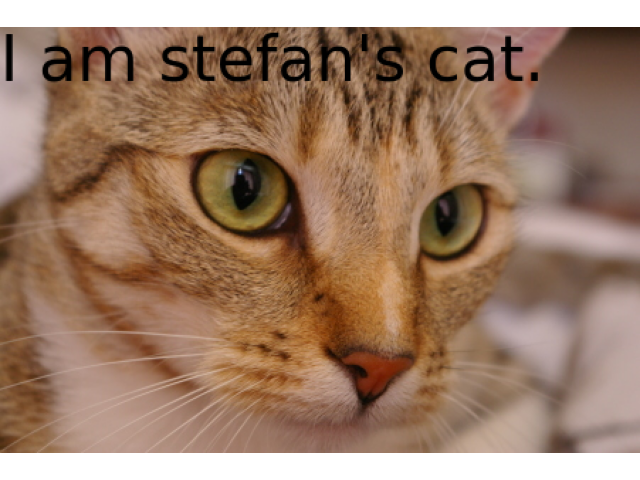# Render text onto an image#

Scikit-image currently doesn’t feature a function that allows you to write text onto an image. However, there is a fairly easy workaround using scikit-image’s optional dependency matplotlib.

```import matplotlib.pyplot as plt
import numpy as np
from skimage import data

img = data.cat()

fig = plt.figure()
fig.figimage(img, resize=True)
fig.text(0, 0.99, "I am stefan's cat.", fontsize=32, va="top")
fig.canvas.draw()
annotated_img = np.asarray(fig.canvas.renderer.buffer_rgba())
plt.close(fig)
```

For the purpose of this example, we can also show the image; however, if one just wants to write onto the image, this step is not necessary.

```fig, ax = plt.subplots()
ax.imshow(annotated_img)
ax.set_axis_off()
ax.set_position([0, 0, 1, 1])
plt.show()
```Total running time of the script: ( 0 minutes 1.069 seconds)

Gallery generated by Sphinx-Gallery